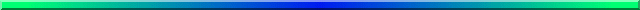# Parametric and Resampling Statistics (cont):The second feature of parametric statistics, with which we are all familiar, is a set of assumptions about normality, homogeneity of variance, and independent errors. I think it is helpful to think of the parametric statistician as sitting there visualizing two populations. One population is the set of all (potential) scores from subjects receiving one treatment, and the other population is the set of all (potential) scores from subjects receiving the other treatment. Our statistician makes the assumption that both of these populations are normal, and both have the same error variance. The only way that is left for them to differ is in their means, and the parametric statistician sets up the null hypothesis that μ1 = μ2. She then proceeds to test that null by asking whether the obtained difference in sample means is likely to arise when the populations have the same means—she has already assumed that they have the same shape and variance.

There are actually two reasons why those parametric assumptions are important. In the first place, they place constraints on our interpretation of the results. If we really do have normality and homoscedasticity, and if we obtain a significant result, then the only sensible interpretation of a rejected null hypothesis is that the population means differ. What could be neater?

The second reason for the assumptions is that we use the characteristics of the populations from which we sample to draw inferences on the basis of the samples. By assuming normality and homoscedasticity, we know a great deal about our sampled populations, and we can use what we know to draw inferences. For example, in a standard t test. we know that if the populations are normal, the sampling distribution of differences between means is also normal. (It would be nearly normal under other conditions, but that is immaterial.) We also know that if the populations have equal variances, we can pool our sample variances, combine that with the sample sizes, and draw a reasonable estimate of the standard error of the distribution of mean differences. We also know that with normal distributions, means and variances are independent. Thus those parameters are important to us, and by making suitable assumptions about them, we can derive a test that is optimal (if the assumptions are valid). So parametric statisticians do really care about those assumptions, even if they speak about the robustness of the test in the presence of assumptions violations. The parameters are at the heart of the test.

For resampling statistics, however, we don't base the test on the population parameters, and thus don't have to make assumptions about them. We work only with the data, and with our expectations about those data if treatments don't have any effect. Of course our conclusions may not be as clear-cut without those assumptions, but that is the price we pay for simplicity and flexibility. And it is often a price worth paying.

Randomization test advocates like Edgington, and Lunneborg don't sit there with visions of populations dancing in their heads. They don't have to worry much about what variances those populations have, or whether they are normal. Those issues are not central to the question they are asking, nor are they central to the logic behind how they answer their question. The resampling folks are extremely proud of pointing out that they don't have to assume normality or homogeneity of variance, but they often forget to point out that that's because they are asking a different question. The parametric tests are asking if the means are different, while the randomization tests are acting as if the treatments have different effects, and I am not using the word "effect" there in its technical statistical sense. It shouldn't come as much of a surprise that when you ask a different question, you need to make different assumptions, and you may get different answers.